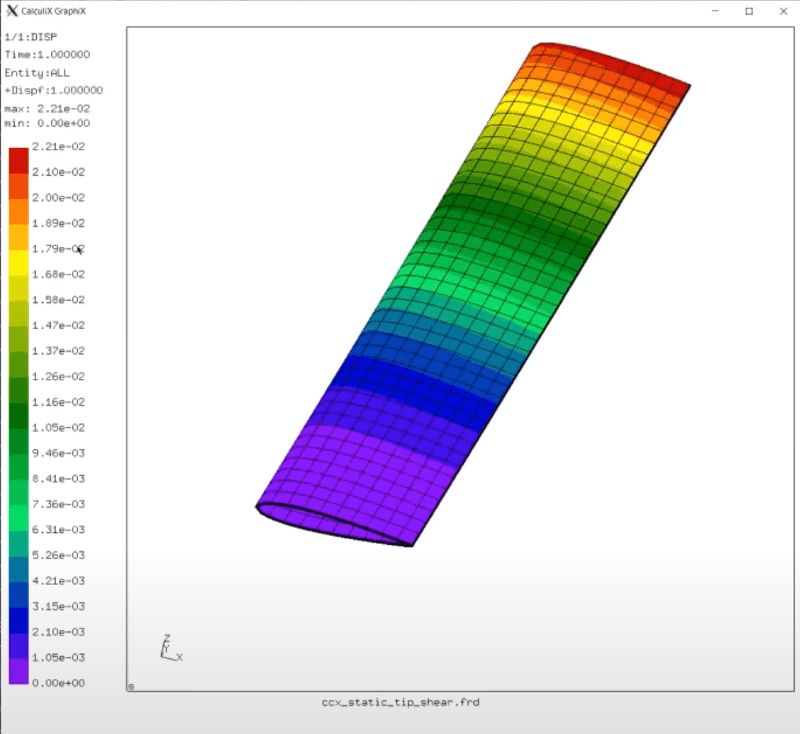Dapta

# Tutorial## Dynamic aeroelastic flutter and divergence analysis with Python and Calculix

We add a dynamic aeroelastic stability analysis to our parametric wing analysis, so that we can calculate critical flutter and divergence flight velocities. We extract mass and stiffness information from our CalculiX model and then solve a complex eigenvalue problem with the help of the Scipy sparse matrix library.## Automated FEM analysis and output processing with Python and CalculiX CrunchiX (ccx)

Automate adding composite material properties to our model, running a static analysis in CalculiX CrunchiX (CCX) and reading & plotting the outputs — so that we can actually run a parametric study…